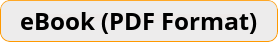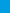# Prepare for the ALEKS Math Test in 7 Days A Quick Study Guide with Two Full-Length ALEKS Math Practice TestsIf you are looking for a quick study book to help you review the most important topics of ALEKS Math Placement Test fast, Prepare for the ALEKS Math Test in 7 Days is just for you.\$19.99 \$13.99
-31% only \$19.99 \$13.99
Rated 5.00 out of 5 based on 1 customer rating

Bulk Orders: Need a license for this book?

### Also included in:

If you are looking for a quick study book to help you review the most important topics of ALEKS Math Placement Test fast, Prepare for the ALEKS Math Test in 7 Days is just for you. In only 7 days you can learn ALEKS Math topics and be well prepared for the test. In this book, you will learn many basic math topics and critical math concepts including integers, mixed numbers, percent, order of operations, ratios, proportions, variables, equations, inequalities, system of equations, polynomials, Pythagorean theorem, quadratics, functions, geometry, and trigonometry.

Prepare for the ALEKS Math Test in 7 Days is designed for those students who need extra practice in a short period of time to help them get the confidence to beat the test. In each chapter of the book, several Math topics are taught in a structured manner with many examples and detailed answers. You only need to follow the step-by-step instruction in the book to learn basic math topics quickly, measure your readiness for the test, and raise your test score.

There are two complete and realistic ALEKS Math Placement Tests in the book to help you learn about the structure of the test and the math topics that you will be tested. Written by ALEKS Math expert and tutors, the content of the book is 100 percent aligned with the 2019 and 2020 test guidelines. Dynamic design and easy-to-follow activities and helpful hints make learning complicated Math topics fun and interesting.

Prepare for the ALEKS Math Test in 7 Days is Ideal for both self–study as well as for classroom usage.

## 1 review for Prepare for the ALEKS Math Test in 7 Days

1.Rated 5 out of 5

I highly recommend this book.

?